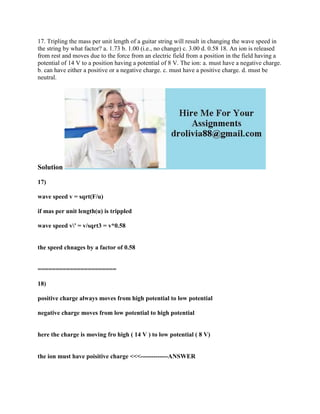Ce diaporama a bien été signalé.
Le téléchargement de votre SlideShare est en cours. ×

# 17- Tripling the mass per unit length of a guitar string will result i.docx

Publicité
Publicité
Publicité
Publicité
Publicité
Publicité
Publicité
Publicité
Publicité
Publicité
PublicitéChargement dans…3
×

## Consultez-les par la suite

1 sur 2 Publicité

# 17- Tripling the mass per unit length of a guitar string will result i.docx

17. Tripling the mass per unit length of a guitar string will result in changing the wave speed in the string by what factor? a. 1.73 b. 1.00 (i.e., no change) c. 3.00 d. 0.58 18. An ion is released from rest and moves due to the force from an electric field from a position in the field having a potential of 14 V to a position having a potential of 8 V. The ion: a. must have a negative charge. b. can have either a positive or a negative charge. c. must have a positive charge. d. must be neutral.
Solution
17)
wave speed v = sqrt(F/u)
if mas per unit length(u) is trippled
wave speed v\' = v/sqrt3 = v*0.58
the speed chnages by a factor of 0.58
======================
18)
positive charge always moves from high potential to low potential
negative charge moves from low potential to high potential
here the charge is moving fro high ( 14 V ) to low potential ( 8 V)
the ion must have poisitive charge <<<-------------ANSWER
.

17. Tripling the mass per unit length of a guitar string will result in changing the wave speed in the string by what factor? a. 1.73 b. 1.00 (i.e., no change) c. 3.00 d. 0.58 18. An ion is released from rest and moves due to the force from an electric field from a position in the field having a potential of 14 V to a position having a potential of 8 V. The ion: a. must have a negative charge. b. can have either a positive or a negative charge. c. must have a positive charge. d. must be neutral.
Solution
17)
wave speed v = sqrt(F/u)
if mas per unit length(u) is trippled
wave speed v\' = v/sqrt3 = v*0.58
the speed chnages by a factor of 0.58
======================
18)
positive charge always moves from high potential to low potential
negative charge moves from low potential to high potential
here the charge is moving fro high ( 14 V ) to low potential ( 8 V)
the ion must have poisitive charge <<<-------------ANSWER
.

Publicité
Publicité

### 17- Tripling the mass per unit length of a guitar string will result i.docx

1. 1. 17. Tripling the mass per unit length of a guitar string will result in changing the wave speed in the string by what factor? a. 1.73 b. 1.00 (i.e., no change) c. 3.00 d. 0.58 18. An ion is released from rest and moves due to the force from an electric field from a position in the field having a potential of 14 V to a position having a potential of 8 V. The ion: a. must have a negative charge. b. can have either a positive or a negative charge. c. must have a positive charge. d. must be neutral. Solution 17) wave speed v = sqrt(F/u) if mas per unit length(u) is trippled wave speed v' = v/sqrt3 = v*0.58 the speed chnages by a factor of 0.58 ====================== 18) positive charge always moves from high potential to low potential negative charge moves from low potential to high potential here the charge is moving fro high ( 14 V ) to low potential ( 8 V) the ion must have poisitive charge <<<-------------ANSWER